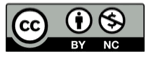# 学习 TikZ (1) 笛卡尔坐标系

2018-09-03## 文档结构

TikZ 的文档结构是

\documentclass{article}
\usepackage{tikz}
\begin{document}
\begin{tikzpicture}
TikZ 命令写在这里，描述一幅图
\end{tikzpicture}
\end{document}


## 命令形式

Latex 命令形式是

\command [可选参数] {参数列表}


\起始动作 (变量) [参数列表] 下一动作 (变量) [参数列表] 下一动作 (变量) [参数列表]…


## 直线

\draw (-1, 0) -- (1, 0);


（落笔到）(--1, 0) ，（画直线到） (1, 0)


\draw (-1, 0) -- (1, 0) -- (0, -1) -- (0, 1);


（落笔到）(-1, 0) ，（画直线到） (1, 0)，（画直线到）(0, -1)，（画直线到） (0, 1)“落笔"后仍然可以"抬笔”，只要中断画线的动作即可，比如:

\draw (-1, 0) -- (1, 0)   (0, -1) -- (0, 1);


（落笔到）(-1, 0) ，（画直线到） (1, 0)，（落笔到）(0, -1) ，（画直线到） (0, 1)## 椭圆和圆

\draw (0, 0) ellipse [x radius=2cm, y radius=1cm];\draw (0, 0) circle [radius=1cm];


## 矩形

\draw (0, 0) rectangle (1, 1);


## 网格线

\draw (0, 0) grid (3, 3);\draw [blue, thick](-0.8, -0.8) grid (3.2, 3.2);  % 蓝格子
\draw [red, thick](1.2, 1.2) grid (5.2, 5.2);  % 红格子
\draw [fill=black](0, 0) [radius=0.1cm] circle;   % 原点## 画图样式

\draw [color = red] (0,0) [radius=1cm] circle;


\draw [red] (0,0) circle (1cm);


• very thin
• thin
• thick
• very thick
• ultra thick

ultra thin 的选项，对于分辨率不高的普通显示器，可能不能正常显示。而且没有 normal 这一选择，如果想表达 thin 和 thick 之间的中间值，要用 semithick.

## 圆弧

\draw (0,0) arc [start angle=-30, end angle=30, x radius=1cm, y radius=3cm];\draw (0,0) arc [start angle=-30, end angle=30, radius=2cm];
\draw (0,0) arc [start angle=-40, end angle=40, radius=3cm];
\draw (0,0) arc [start angle=-50, end angle=50, radius=4cm];## 裁剪

\clip (0, 0) circle (1.9);
\draw (-1.5, -1.5) rectangle (1.5, 1.5);\clip 命令还有一个可选参数 [draw] ，启用后，将同时画出裁剪的边界，比如

\clip[draw] (0, 0) circle (1.9);
\draw (-1.5, -1.5) rectangle (1.5, 1.5);(未完待续。。。。。)

## 精彩评论